Ch.14 - Chemical EquilibriumWorksheetSee all chapters
 Ch.1 - Intro to General Chemistry 2hrs & 53mins 0% complete Worksheet Ch.2 - Atoms & Elements 2hrs & 49mins 0% complete Worksheet Ch.3 - Chemical Reactions 3hrs & 25mins 0% complete Worksheet BONUS: Lab Techniques and Procedures 1hr & 38mins 0% complete Worksheet BONUS: Mathematical Operations and Functions 47mins 0% complete Worksheet Ch.4 - Chemical Quantities & Aqueous Reactions 3hrs & 55mins 0% complete Worksheet Ch.5 - Gases 3hrs & 47mins 0% complete Worksheet Ch.6 - Thermochemistry 2hrs & 28mins 0% complete Worksheet Ch.7 - Quantum Mechanics 2hrs & 35mins 0% complete Worksheet Ch.8 - Periodic Properties of the Elements 1hr & 57mins 0% complete Worksheet Ch.9 - Bonding & Molecular Structure 2hrs & 5mins 0% complete Worksheet Ch.10 - Molecular Shapes & Valence Bond Theory 1hr & 31mins 0% complete Worksheet Ch.11 - Liquids, Solids & Intermolecular Forces 3hrs & 40mins 0% complete Worksheet Ch.12 - Solutions 2hrs & 17mins 0% complete Worksheet Ch.13 - Chemical Kinetics 2hrs & 22mins 0% complete Worksheet Ch.14 - Chemical Equilibrium 2hrs & 26mins 0% complete Worksheet Ch.15 - Acid and Base Equilibrium 4hrs & 42mins 0% complete Worksheet Ch.16 - Aqueous Equilibrium 3hrs & 48mins 0% complete Worksheet Ch. 17 - Chemical Thermodynamics 1hr & 44mins 0% complete Worksheet Ch.18 - Electrochemistry 2hrs & 58mins 0% complete Worksheet Ch.19 - Nuclear Chemistry 1hr & 33mins 0% complete Worksheet Ch.20 - Organic Chemistry 3hrs 0% complete Worksheet Ch.22 - Chemistry of the Nonmetals 2hrs & 1min 0% complete Worksheet Ch.23 - Transition Metals and Coordination Compounds 1hr & 54mins 0% complete Worksheet

# Chemical Equilibrium

See all sections
Sections
Chemical Equilibrium
ICE Chart
Le Chatelier's Principle
The Reaction Quotient
Equilibrium Expressions

Most chemical reactions never go to completion where all the reactants are converted into products. Instead these reactions reach a chemical equilibrium

###### Chemistry & Equilibrium

Concept #1: Understanding Chemical Equilibrium.

Transcript

Hey guys! In this brand new video, we're going to take a look at the Equilibrium State and figure out when exactly do some reactions reach equilibrium.
Now first we're going to say that most chemical reactions never go to completion. When we say the word completion all that really means is that our reactants do not completely convert into products. Our reaction that goes to completion, every amount of reactant that's there gets broken down in order to build up to make our products. But we're going to say that this doesn't happen with all chemical reactions. So there's going to be some amount of reactant left. Now that amount could be a very small amount or could be a very large amount. It all depends on the chemical reaction. Now since our reactant amount is always going to be some amount, we're going to say reaction concentrations do not go down to 0. Now we're going to say instead these chemical reactions reach a state of chemical equilibrium in which the reaction moves in the forward and the reverse direction. Remember we talked about this, we said that these reactions have double arrows, one going in the forward one going in the reverse. And we're going to say because they can go in the forward and the reverse direction, they're called reversible reactions.
Here we have a typical reaction, we have reactant A going in the forward direction to give us product B. And just realize we're going in the forward direction here with this forward facing arrow and we use K1 to signify this forward direction. Now on the same way the reaction could go in the opposite direction where some of our product actually breaks down itself in order to rebuilt some of the reactant that was lost. When we’re going in the reverse direction we use K-1. The minus signifies that we're going in the reverse direction. Now if we're going to look at this plot here, you can see on this plot that our blue line represents our reactant A, our red line represents our product B. And what you should take from this chart is this, we're going to be losing reactants, so we lose reactants to make products. So that's the most common thing that we have to take from this image. And what you should realize here is eventually product amount will stop increasing and reactant amount will stop decreasing and they'll reach a plateau for both of them. So there amounts were going to be held constant after a while. It's at this 5 minute mark where they become constant in their concentrations. So we're going to say equilibrium happens at that exact moment. So that's how we're able to tell a reaction has reached chemical equilibrium. The reactant and the product concentrations or amounts have been held constant. Now reactions are still going on on a molecular level but for every amount of reactant we lose, it's okay because our products are going in their reverse direction to make up for what we just lost. So in that way their levels maintain a same number overtime. So we're going to say once our amounts plateau that's when equilibrium is reached.
Now knowing that, I want you guys to take a look at this practice question on the bottom and try your best to answer it on your own. Once you figured out the answer, come back on our next video and click on the explanation button after answering and see if your answer matches up with my own. Good luck on this question guys!

A reaction reaches equilibrium once the rate of the forward reaction equals the rate of the reverse reaction. Once at equilibrium there is no net change in the concentration of reactants or products.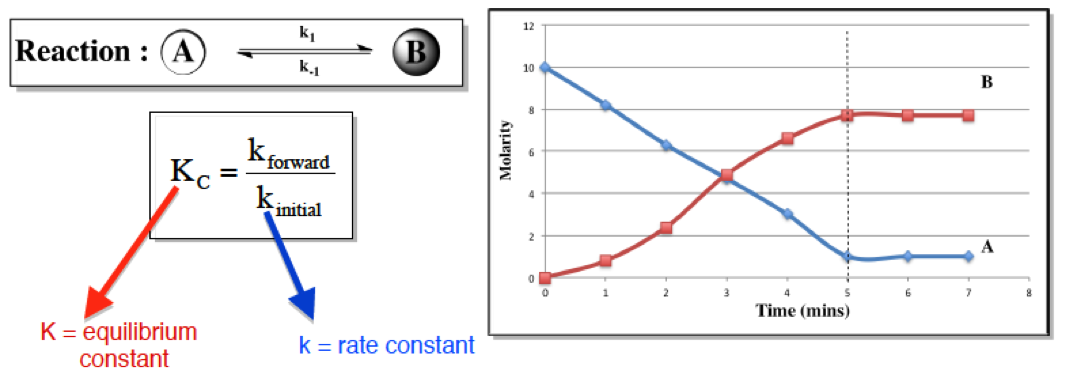Whereas the rate constant, k, is affected by factors dealing with the rate of reaction, the equilibrium constant, K, is affected by only temperature.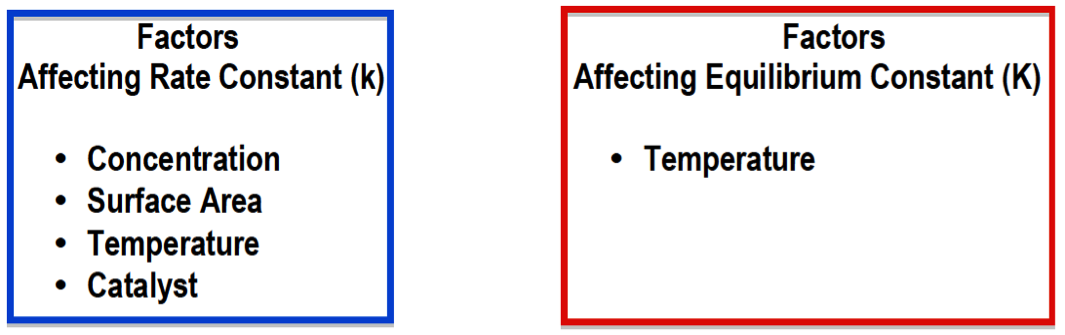Practice: Which one of the following statements does not describe the equilibrium state?

a. While at equilibrium, a dynamic process is still occurring.

b. The concentration of the reactants is equal to the concentration of the products.

c. The concentration of the reactants and products reach a constant level.

d. At equilibrium, the net concentration of all species is not changing.

e. All are true.

###### The Equilibrium Constant K

Associated with any reaction at equilibrium is the equilibrium constant K. Its numerical value determines if reactants or products are more greatly favored within a reaction.

Concept #2: Understanding the Equilibrium Constant.

Transcript

Hey guys! In this brand new video, we're going to take a look at the Equilibrium Constant.
Now we're going to say that the variable we use to illustrate our equilibrium constant is the variable K. And we're going to say K is a number equal to the ratio of products to reactants at a given temperature. Why do I say at a given temperature? Because we're going to say that temperature directly affects our equilibrium constant K. Increasing the temperature will increase our K value, decreasing our temperature will decrease our K value. Now we're going to say it's a ratio of products to reactants so we're going to say that here K = products/reactants. Now we're going to say that K is important because its magnitude tells us how far to the left or to the right our chemical reaction is at a given temperature. So we're going to say if K is greater than 1 then products are favored over reactants. And think about it, this makes sense because we said K = products/reactants so let's say our products are 10 and our reactants are 1, K = 10 definitely greater than 1. We're going to say when products are favored over our reactants that mean we're making more products. How do we make more products? By going in the forward direction. So the forward direction would be favored. Now in the opposite way, if K is less than 1, so let's say we have in this case products are 1 but our reactants are 10, we'd have a K less than 1. So in this case, if K is less than 1 then reactants are favored over products. Which means our reaction is heading in the reverse direction. So the reverse direction would be favored. So just remember these 2 differences, when K is greater than 1, when K is less than 1. Now what if we say K was equal to 1? We know that this is products/reactants so that means our products and reactants would have to be equal, let's say they're both 10. 10/10 is 1. So when K = 1 we're going to say both our reactant and our product amounts are equal to one another. Now we're going to say the equilibrium constant K takes into account all the stats of matter except 2, it doesn't look at solids and it doesn't look at liquids, it ignores those 2 states for matter.

The equilibrium constant K is a ratio of products to reactants. It only deals with gaseous or aqueous compounds.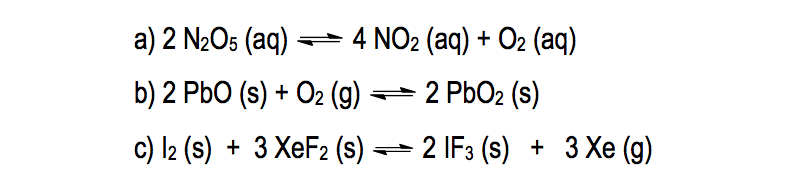Example #1: Write the equilibrium expression for the following reaction:

Transcript

So it looks at it only cares about really gases and also aqueous. Aqueous just means that the solvent is water, so a compound is dissolved in water. It would be aqueous.
Now knowing this, let's take a look at the following Example. It says:
Write the equilibrium expression for the following reaction.
Here we have 2 and 2 N2O5 (aq) gives us 4 NO2 (aq) + O2 (aq). It's important to look at the phases because remember if it's a solid or a liquid we ignore it. Now we're going to say here K = products/reactants. So we're going to have our products as NO2. And remember you have to use the coefficients in these calculations. The number in front of NO2 is a 4, so that 4 will become the power, so it's going to be (NO2)4 * O2, O2. just has a coefficient of 1 in front of it which we don't have to show, divide it by N2O5, again the coefficient in front of N2O5 is 2 so that becomes a power.

K = products = (NO2)4 * (O2)
reactants (N2O5)2

So we would say that this represents our equilibrium expression or our equilibrium equation. Same thing, equilibrium expression, equilibrium equation.
Now that we've seen this one, let's look at B. For B we're going to say here, we're going to ignore this compound because it's a solid and we're going to ignore this compound because it's a solid. Here we're going to say K = products/, like before. But here's the thing, we're going to say we don't have any products available but you have to put something on top. We're going to say it's equal to 1. Solids and liquids are ignored and in place of them we're going to put 1. We're going to say this because things such as pressured don't really affect solids and liquids like they do gases and aqueous compounds, that's why they're equal to 1 because their number’s being held constant. So we're going to replace solids and liquids with the number 1. And on the bottom we'd have O2.

K = products = 1
reactants (O2)1

Now different way your professor could give you this is, we know there's a 1 here so this could also be or O2 inverse 1. So be aware of both type of answers. Both are correct, both are saying the same thing. Inverse 1 just means 1 over whatever it is.

K = products = 1 or O2-1
reactants (O2)1

For the next one, again we ignore solids. The only thing that we don't ignore is this gas. So here K = products/reactants, so it just equal Xe3 because of the 3 over 1. But we don’t need to put the 1 because anything over 1 is the same exact thing. So that would be our equilibrium expression.
K = (Xe)3
So A was pretty simple but remember the difference is in B and C, we ignore the solids and the liquids. Now that you've seen this, I want you guys to attempt to answer question that follows this one, the practice 1. Here I want you to tell me who's greater in amount, is it products or is it reactants? From that you have to remember what do we say about K? When it's greater than 1 who's favored? When it's less than 1 who's favored? When it's equal to 1 who's favored? Remembering that would be a great way for you to approach this problem. Good luck guys!

Practice: State which is greater in amount: reactants or products, based on the given equilibrium constant, K.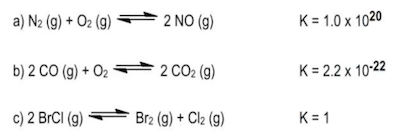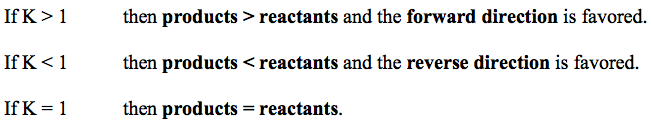Practice: The decomposition of nitrogen monoxide can be achieved under high temperatures to create the products of nitrogen and oxygen gas.

6 NO(aq) ⇌ 3 N2(aq) + 3 O2(aq)

a) What is the equilibrium equation for the reaction above?

b) What is the equilibrium expression for the reverse reaction.

Practice: The equilibrium constant, K, for 2 NO (g) + O2 (g) ⇌ 2 NO2 (g) is 6.9 x 102

What is the [NO] in an equilibrium mixture of gaseous NO, O2, and NO2 at 500 K that contains 1.5 x 10 –2 M O2 and 4.3 x 10 –3 M NO2?

###### Kp and Kc

When dealing with equilibrium units in terms of pressure the equilibrium constant of Kp must be used, but if the equilibrium units are in molarity then we must use the equilibrium constant of Kc

Concept #3: The Different Types of Equilibrium Constants.

Transcript

Hey guys in this new video we're going to take a look at different types of the Equilibrium Constants.
Now one thing that we're going to realize by going through Chemistry 1 and Chemistry 2 is that "K" is a very very popular variable. We have it everywhere. We have a rate constant K. We have actually a K for acids and bases, we call them Ka and Kb. Ka is called our acid association constant, whereas Kb is called our bases association constant. Now we're talking about different types of Equilibrium Constants. So "K" is really really popular when it comes to calculations in Chemistry.
Now, we've talked about the equilibrium constant up to this point. we said that it's equal to products over reactants. And we should realize this that we have different types of Equilibrium Constants based on the units that we're using. Now we're going to say when dealing with gases we usually use the equilibrium constant Kp. We're going to say Kp uses the partial pressure unit of atmospheres.
And were going to say that when dealing with aqueous solutes, remember aqueous means that water is dissolving bicompound. So when we're using aqueous solutes, we tend to use the equilibrium constant Kc. Now Kc uses the concentration unit of molarity and remember molarity is moles over liters. And also remember molarity is the same thing as concentration and the same thing as solubility.
So, we've seen that we have Kp and Kc now. And we're going to say that these two are related together by this equation. Kp = Kc (RT) ∆n. Here we're going to say that R is called our Gas Constant and it equals 0.08206 liters times atmospheres over moles times K. Now remember there's another R variable out there it’s 8.314 joules over moles times K. But we only use that one when we're dealing with energy, velocity or speed. In this case we are not dealing with energy, velocity or speed. We are dealing with Equilibrium Concentrations. So that's where we're using this particular R. We're going to say that T represents our Temperature in Kelvin. And there we're going to say most importantly is our ∆n exponent. ∆n equals moles of gas as products minus moles of gas. Again remember gas as reactants. So we've started out with the broad idea of an Equilibrium Constant K but now we've delve than deeper to Kp and Kc.

Kp and Kc are related to o